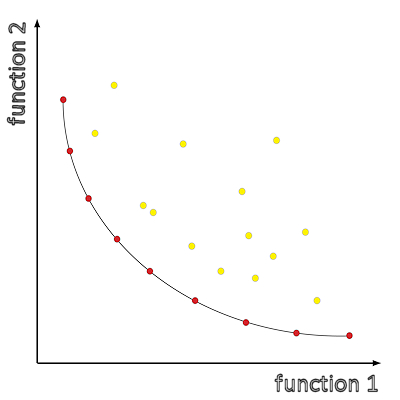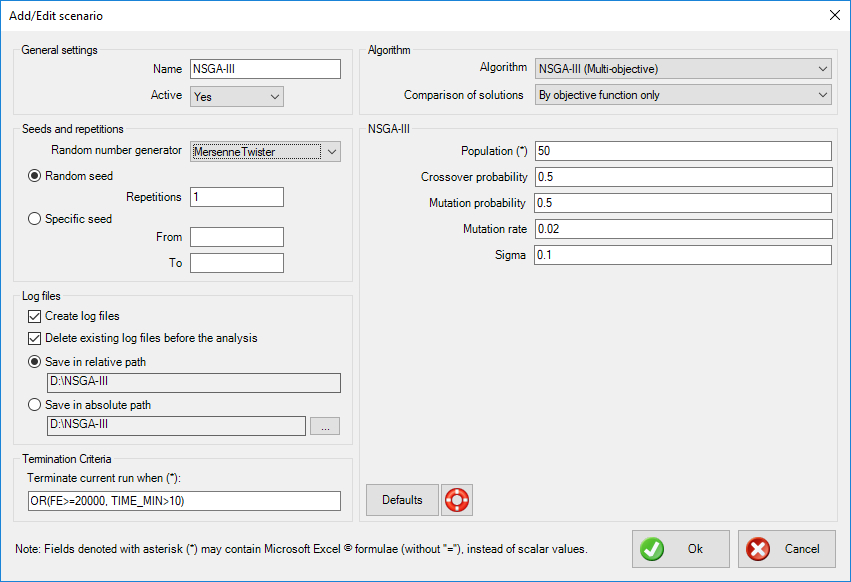## Introduction

Multi-objective optimization (also known as multi-criteria optimization or Pareto optimization) is concerned with mathematical optimization problems involving more than one objective function which are to be optimized simultaneously. Multi-objective optimization has been applied in many fields of science, including engineering, economics and logistics where decisions need to be taken in the presence of trade-offs between two or more conflicting objectives.

Maximizing performance whilst minimizing fuel consumption of a vehicle is an example of multi-objective optimization.

For non-trivial problems, no single solution exists that simultaneously optimizes each objective. Usually, a number of so-called Pareto-optimal solutions exists, forming Pareto fronts, which are alternative solutions to the problem. The optimality in this case is defined based on the context of dominance; a Pareto-optimal solution is nondominated, i.e., for this solution, none of the objective functions can be improved in value without degrading some of the other objective values.

xlOptimizer fully implements NSGA-III, a well-known multi-objective algorithm 

## How does it work?

NSGA-III is a real-coded genetic algorithm, i.e. it does not use binary representation of the chromosomes. A number of solutions form the initial population, which is extended to include a number of offspring solutions (created by crossover) and a number of mutant solutions (created by mutation of the population). These form the extended population, which is sorted, based on dominance, and categorized by rank (corresponding to each front). The extended population is then truncated to its original size and the process is repeated.

## Options in xlOptimizer add-in

The following options are available in xlOptimizer add-in. Note that whenever an asterisk (*) is indicated in a text field, this means that the field accepts a formula rather than a certain value. The formula is identical to Microsoft Excel's formulas, without the preceding equal sign '='. Also, the function arguments are always separated by comma ',' while the dot '.' is used as a decimal point.#### General settings

• Name: the name of the scenario to be run. It should be descriptive but also concise, as a folder with the same name will be (optionally) created, which will hold the log files for further statistical analysis.
• Active: select whether the scenario is active or not. If it is active, it will be run in sequence with the other active scenaria. In the opposite case, it will be ignored. This is very helpful when you experiment with settings.

#### Seeds and repetitions

Metaheuristic algorithms are based on random number generators. These are algorithms that produce a different sequence of random numbers for each seed they begin with. A seed is just an integer, and a different seed will produce a different evolution history with a different outcome. Robust metaheuristic algorithms should, on average, perform the same no matter what the seed is.

• Random number generator: select the random number generator to be used. The Mersenne Twister is default. The following options are available:
• NumericalRecipes: Numerical Recipes'  long period random number generator of L’Ecuyer with Bays-Durham shuffle and added safeguards
• SystemRandomSource: Wraps the .NET System.Random to provide thread-safety
• CryptoRandomSource: Wraps the .NET RNGCryptoServiceProvider
• MersenneTwister: Mersenne Twister 19937 generator
• Xorshift: Multiply-with-carry XOR-shift generator
• Mcg31m1: Multiplicative congruential generator using a modulus of 2^31-1 and a multiplier of 1132489760
• Mcg59: Multiplicative congruential generator using a modulus of 2^59 and a multiplier of 13^13
• WH1982: Wichmann-Hill's 1982 combined multiplicative congruential generator
• WH2006: Wichmann-Hill's 2006 combined multiplicative congruential generator
• Mrg32k3a: 32-bit combined multiple recursive generator with 2 components of order 3
• Palf: Parallel Additive Lagged Fibonacci generator
• Random repetitions: use this option if you wish the program to select random seeds for every run. Also, select the number of repetitions. If you wish to perform a statistical analysis of the results, a sample of 30 runs should be sufficient.
• Specific seed: use this option if you wish to use a selected range of integers as seeds. Note that seeds are usually large negative integers.

#### Log files

• Create log files: select whether you wish to create a log file for each run. This is imperative if you wish to statistically analyze the results. If you are only interested in the best result out of a certain number of runs, disabling this will increase performance (somewhat).
• Delete existing log files before the analysis: select this option if you want the program to delete all existing (old) log files (with the extension ".output") in the specific folder, before the analysis is performed.
• Save in relative path: select this option to create and use a folder relative to the spreadsheet's path. The name of the folder is controlled in the General Settings > Name.
• Save in absolute path: select this option to create and use a custom folder. Press the corresponding button with the ellipses "..." to select the folder.

#### Termination criteria

Each scenario needs a specific set of termination criteria. You can use an excel-like expression with the following variables (case insensitive):

• 'BEST_1', 'BEST_2', etc represent the best value of the objective function 1, 2, etc in the population.
• 'AVERAGE_1', 'AVERAGE_2', etc represent the average value of the objective function 1, 2, etc in the population.
• 'WORST_1', 'WORST_2', etc represent the worst value of the objective function 1, 2, etc in the population.
• 'MIN_1', 'MIN_2', etc represent the minimum value of the objective function 1, 2, etc in the population.
• 'MAX_1', 'MAX_2', etc represent the maximum value of the objective function 1, 2, etc in the population.
• 'FE' represents the function evaluations so far.
• 'TIME_MIN' represents the elapsed time (in minutes) since the beginning of the run.
• 'BEST_REMAINS_FE' represents the number of the last function evaluations during which the best solution has not been improved.

The default formula is 'OR(FE>=20000, TIME_MIN>10)' which means that the run is terminated when the number of function evaluations is more than 20000 or the run has lasted more than 10 minutes.

#### Algorithm

• Algorithm: select the algorithm to be used in the specific scenario. In this case 'NSGA-III (Multi-Objective)' is selected.
• Comparison of solutions: select whether feasibility (i.e. the fact that a specific solution does not violate any constraints) is used in the comparison between solutions. The following options are available:
• By objective function only: feasibility is ignored, and only the objective function (potentially including penalties from constraint violations) is used to compare solutions.
• By objective function and feasibility: the objective function (potentially including penalties from constraint violations) is used to compare solutions, but in the end, a feasible solution is always preferred to an infeasible one.

## NSGA-III (Multi-Objective)

The following options are specific to NSGA-III.

#### Population

Enter a formula for the calculation of the population size. You can use an excel-like expression with the following variables (case insensitive):

• 'VARS' represents the number of variables (i.e. the dimensionality of the problem).

The default setting is '80', which is a good choice for most problems.

#### Crossover probability

Select an appropriate value for the crossover probability. This signifies the percentage of population which will be added temporarily as offspring in the extended population by means of crossover of existing members. The default setting is 0.5.

#### Mutation probability

Select an appropriate value for the mutation probability. This signifies the percentage of population which will be added temporarily as mutated members in the extended population. The default setting is 0.5..

#### Mutation rate

Select an appropriate value for the mutation rate. This controls the percentage of design variables in the chromosome that will be mutated (at least one design variable per mutant is mutated, however).

#### Sigma

Select an appropriate value for the sigma coefficient. This controls the mutation magnitude per variable. Sigma is multiplied by a random variable following standard normal distribution.